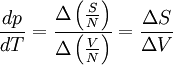# Clausius-Clapeyron relation$\frac{dp}{dT} = \frac{\Delta \left( \frac{S}{N}\right)}{\Delta \left( \frac{V}{N}\right)} = \frac{\Delta S}{\Delta V}$